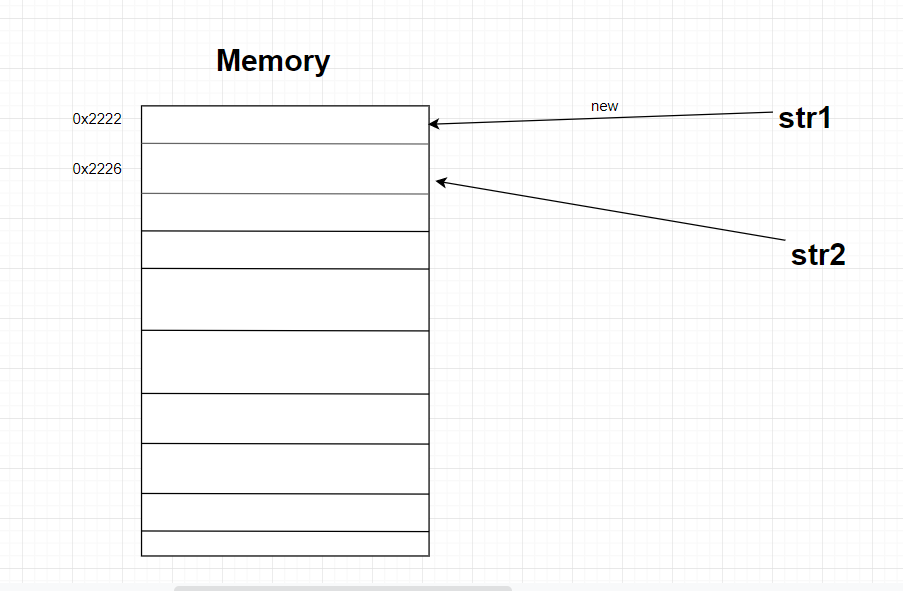# Java equals() Method Example

Java equals() method and the “==” operator are both used to compare objects for equality. However, they are doing the checking in a very different way, generating different results.The main difference between them is that “==” checks if both objects point to the same memory location and equals() evaluates to the comparison of the actual values contained in the objects.

An example would give you even more clue:

Animal.java

```public class Animal {
private String name;
private int age;

public Animal (String n, int a) {
this.name = n;
this.age = a;
}
}
```

EqualsDemo.java

```public class EqualsDemo {
public static void main(String[] args) {
Animal animal1 = new Animal("Vic", 4);
Animal animal2 = new Animal("Vic", 4);

if (animal1 == animal2)
System.out.println("These objects are equal.");
else
System.out.println("These objects are not equal.");
}
}
```

What do you think will be printed out?

Output:

```These objects are not equal.
```

Even though both objects are instances of the same Class and contain the same values, they are do not refer to the same object. Whenever you type the new keyword, automatically it creates a new Object reference. When we are creating two objects using the new keyword, they are not the same, not even if they contain the same values. They point to different memory locations.

## Comparing strings using the equals() method and the “==” operator

EqualsDemo.java

```public class EqualsDemo {
public static void main(String[] args) {
String str1 = "First string";
String str2 = "First string";

if (str1 == str2)
System.out.println("Equal");
else
System.out.println("Not equal");
}
}
```

What do you think will be printed to the screen?

Output:

`Equal`

If you said Equal, then you would have been correct. When the strings contain the same cont

`Not equal`

ent, they point to the same memory location.

Now let’s do th exact same example as above but using the new keyword instead.

## Creating same-content Strings created with a new keyword

EqualsDemo.java

```public class EqualsDemo {
public static void main(String[] args) {
String str1 = new String("First string");
String str2 = new String("First string");

if (str1 == str2)
System.out.println("Equal");
else
System.out.println("Not equal");
}
}
```

What do you think would be printed now?

Output:

`Not equal`

The reason Not equal is printed is because, as I pointed above, when you create objects using the new keyword, you create a new Pointer that points to its own memory location.This is a visual example. The memory locations are just made up. But you can see from the example that when str1 is created and str2 is created, they point to different memory locations. So when you use the == operator to compare them, you will get false no matter what.

## Overriding equals() method to fit out criteria

Let’s say you wanted to call .equals() on two objects and if they contain the same name and age, it should return true.

Animal.java

```public class Animal {
private String name;
private int age;

public Animal (String n, int a) {
this.name = n;
this.age = a;
}

public String getName() {
return this.name;
}

public int getAge() {
return this.age;
}

public boolean equals(Animal a) {
if (this.name.equals(a.getName()) && this.age == a.getAge())
return true;
else
return false;

}
}
```

EqualsDemo.java

```public class EqualsDemo {
public static void main(String[] args) {
Animal animal1 = new Animal("Vic", 4);
Animal animal2 = new Animal("Vic", 4);

if (animal1.equals(animal2))
System.out.println("These objects are equal.");
else
System.out.println("Not equal");
}
}
```

Output:

`These objects are equal.`

We override the equals method in the Animal class so it fits our own criteria. If we didn’t override it and simply called the equals method on the two objects, it wouldn’t return true.

## Calling equals() on Strings

EqualsDemo.java

```public class EqualsDemo {
public static void main(String[] args) {
String str1 = "str";
String str2 = "str";

if(str1.equals(str2)) {
System.out.println("equal");
}
else {
System.out.println("not equal");
}
}
}```

Output

`equal`

When calling equals() on strings, it checks every character if it is the same in both strings. Meaning, you should always use equals when comparing strings and not ==.

## Calling equals on Strings created with a new keyword

EqualsDemo.java

```public class EqualsDemo {
public static void main(String[] args) {
String str1 = new String("str");
String str2 = new String("str");

if(str1.equals(str2)) {
System.out.println("equal");
}
else {
System.out.println("not equal");
}
}
}
```

Output:

`equal`

As you can see, it doesn’t matter whether the two objects (strings) point to different memory locations when using equals. If the content in the two strings is the same, then it returns true.

## Takeaway

You should always use .equals() and not == when comparing strings.